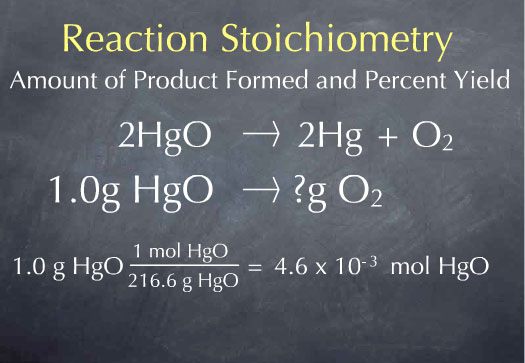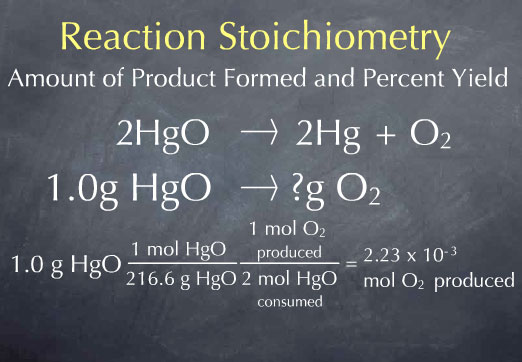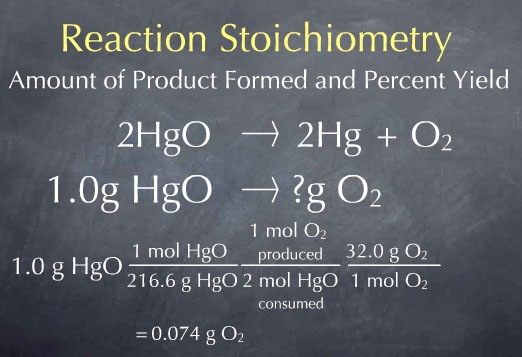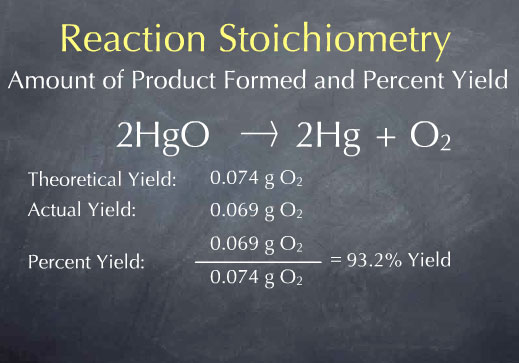# Stoichiometry Tutorials: The Stoichiometry of Product Formation and Percent Yield

(from a complete OLI stoichiometry course)

In another tutorial, we considered the amount of reactant consumed by a chemical reaction. Here, we'll consider the amount of product formed by a reaction.

### Product Formation and Percent Yield Movie Text

One of the things reaction stoichiometry allows us to do is determine the amount of product formed from a given amount of reactant. As an example, consider the reaction that Joseph Priestley used to discover oxygen.

HgO → Hg + O2

Priestly heated up HgO, which produced Hg and O2 gas. Preistley captured the oxygen gas being released by this reaction and studied its properties. Here, we'll consider the amount of oxygen Preistley should have obtained from a given amount of HgO.

We begin by balancing the chemical reaction. We can do this by putting a 2 in front of HgO and Hg, since that leads to 2 Hg and 2 O atoms on both sides of the reaction. These coefficients 2 and 1 are often called stoichiometric coefficients since they capture the reaction stoichiometry: 2 HgO molecules react to form 2 Hg atoms and one oxygen molecule.

2 HgO → 2 Hg + O2

Suppose Priestly had heated up 1.0 gram of HgO. How many grams of oxygen would we expect to be produced? Our starting point is one gram of mercuric oxide. Reactions occur on the molecule scale, so we first need to convert from the macroscopic scale of grams of mercuric oxide to the microscopic scale of the number of molecules of mercuric oxide. To do this, we use the molecular weight.There is 1 mole of mercuric oxide for every 216.6 grams of mercuric oxide, so we have 4.6 x 10-3 moles of mercuric oxide. We now know the number of HgO molecules present in 1.0 gram of sample. From the stoichiometric coefficients of the reaction, we can construct the ratio (1 mol O2 produced)/(2 mol HgO consumed), which says that one molecule of oxygen is produced for every 2 molecules of mercuric oxide that are consumed.Multiplying by this ratio gives us 2.2 x 10-3 moles of oxygen produced. We now know the amount of oxygen produced in terms of the number of oxygen molecules, which is at the molecular scale. In the laboratory, we would measure the amount of the substance in grams. To get to this laboratory scale, we multiply by the molecular weight of oxygen, 32.0 grams per mole, to get 0.074 grams of oxygen. This is the answer to our original question: for every 1.0 gram of HgO that Priestly heated up, he should have produced 0.074 grams of oxygen.We call 0.074 g O2 the theoretical yield, and it is the maximum amount of product that can be formed. In many cases, the amount of product obtained is considerably smaller. For instance, there may be impurities present in the reactants, the reaction may not go to completion, or some of the product may be lost and not captured by the experimenter. Letâ€™s say that Priestly actually captured 0.069 grams of oxygen from 1.0 g of HgO. We call 0.069 g O2 the actual yield.The percent yield is the ratio between the actual and theoretical yield, expressed as a percent. The theoretical yield is the maximum amount of product we can obtain, and the percent yield tells how much of this was actually obtained.

The content above has been converted from Adobe Flash Player and may not display correctly.